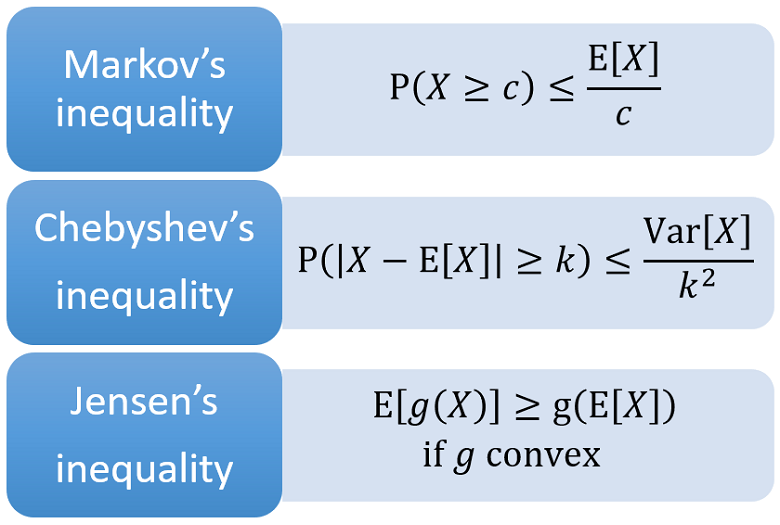StatLect

# Markov's inequality

Markov's inequality is a probabilistic inequality. It provides an upper bound to the probability that the realization of a random variable exceeds a given threshold.## Statement

The proposition below formally states the inequality.

Proposition Letbe an integrable random variable defined on a sample space. Letfor all(i.e.,is a positive random variable). Let(i.e.,is a strictly positive real number). Then, the following inequality, called Markov's inequality, holds:Reading and understanding the proof of Markov's inequality is highly recommended because it is an interesting application of many elementary properties of the expected value.

Proof

First note thatwhereis the indicator of the eventandis the indicator of the event. As a consequence, we can writeNow, note thatis a positive random variable and that the expected value of a positive random variable is positive:Therefore,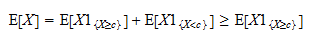Now, note that the random variableis smaller than the random variablefor any:because, trivially,is always smaller thanwhen the indicatoris not zero. Thus, by an elementary property of the expected value, we have that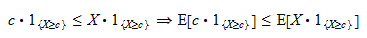Furthermore, by using the linearity of the expected value and the fact that the expected value of an indicator is equal to the probability of the event it indicates, we obtain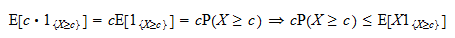The above inequalities can be put together: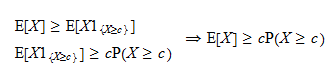Finally, sinceis strictly positive we can divide both sides of the right-hand inequality to obtain Markov's inequality:This property also holds whenalmost surely (in other words, there exists a zero-probability eventsuch that).

## Example

Suppose that an individual is extracted at random from a population of individuals having an average yearly income of \$40,000.

What is the probability that the extracted individual's income is greater than \$200,000?

In the absence of more information about the distribution of income, we can use Markov's inequality to calculate an upper bound to this probability:Therefore, the probability of extracting an individual having an income greater than \$200,000 is less than.

## Important applications

Markov's inequality has several applications in probability and statistics.

For example, it is used:

## Solved exercises

Below you can find some exercises with explained solutions.

### Exercise 1

Letbe a positive random variable whose expected value isFind a lower bound to the probabilitySolution

First of all, we need to use the formula for the probability of a complement:Now, we can use Markov's inequality:Multiplying both sides of the inequality by, we obtainAddingto both sides of the inequality, we obtain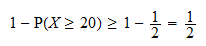Thus, the lower bound is### Exercise 2

Letbe a random variable such that the expected valueexists and is finite.

Use the latter expected value to derive an upper bound to the tail probabilitywhereis a positive constant.

Solution

By Markov's inequality, we have## Other inequalities

If you like this page, StatLect has other pages on probabilistic inequalities: